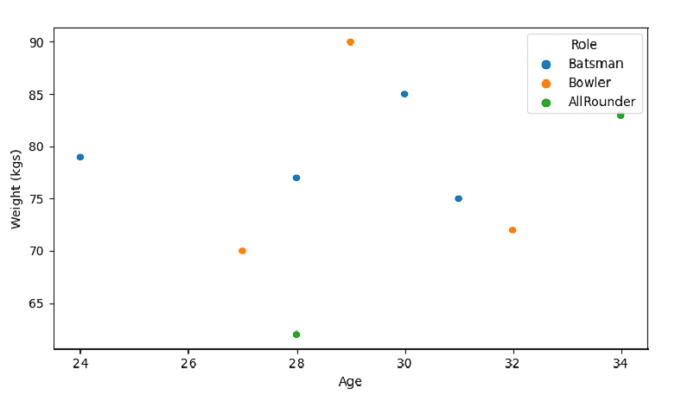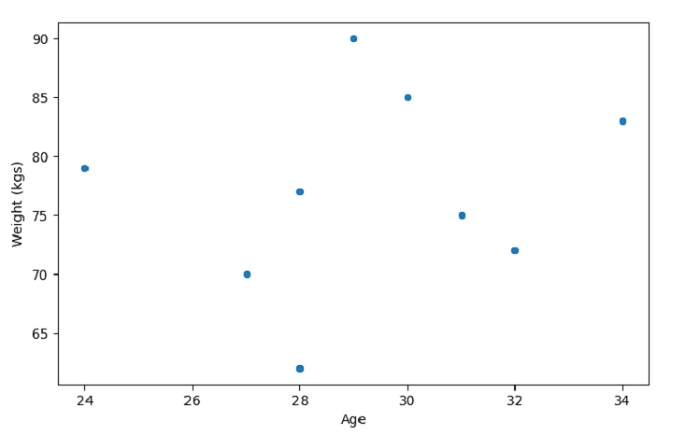# Create a Scatter Plot with SeaBorn â€“ Python Pandas

SactterPlot in Seaborn is used to draw a scatter plot with possibility of several semantic groupings. The seaborn.scatterplot() is used for this.

Let’s say the following is our dataset in the form of a CSV file − Cricketers.csv

At first, import the required 3 libraries −

import seaborn as sb
import pandas as pd
import matplotlib.pyplot as plt

Load data from a CSV file into a Pandas DataFrame −

dataFrame = pd.read_csv("C:\Users\amit_\Desktop\Cricketers.csv")


Plotting scatterplot with Age and Weight (kgs). The hue parameter set as "Role" −

sb.scatterplot(dataFrame['Age'],dataFrame['Weight'], hue=dataFrame['Role'])

## Example

Following is the code −

import seaborn as sb
import pandas as pd
import matplotlib.pyplot as plt

# Load data from a CSV file into a Pandas DataFrame:

# plotting scatterplot with Age and Weight (kgs)
# hue parameter set as "Role"
sb.scatterplot(dataFrame['Age'],dataFrame['Weight'], hue=dataFrame['Role'])

plt.ylabel("Weight (kgs)")
plt.show()

## Output

This will produce the following example −## Example

Let us see another example, wherein we haven’t set the hue parameter. Following is the code −

import seaborn as sb
import pandas as pd
import matplotlib.pyplot as plt

# Load data from a CSV file into a Pandas DataFrame:

# plotting scatterplot with Age and Weight
# weight in kgs
sb.scatterplot(dataFrame['Age'],dataFrame['Weight'])

plt.ylabel("Weight (kgs)")
plt.show()

## Output

This will produce the following output −